#### Calc

##### Description

Calc[exp] performs several basic simplifications. Calc[exp] is the same as DotSimplify[DiracSimplify[Contract[DiracSimplify[Explicit[ SUNSimplify[Trick[exp], Explicit->False] ]]]]]

#####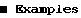This calculates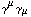in 4 dimensions and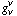in D dimensions.

``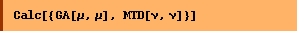``
`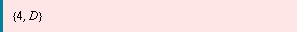`

This simplifies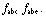``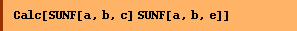``
`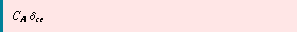`
``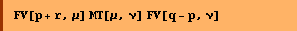``
`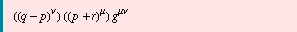`
``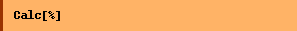``
`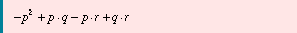`
``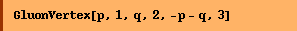``
`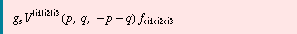`
``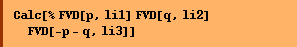``
`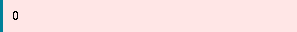`

Converted from the Mathematica notebook Calc.nb